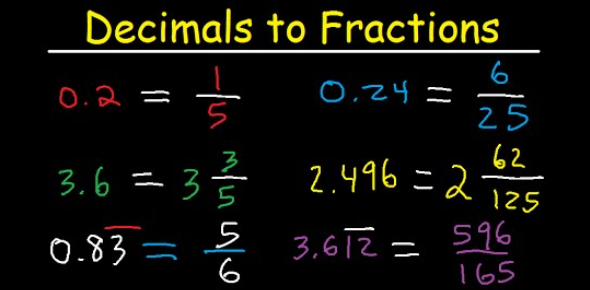# Can You Pass This Decimal To Fraction Quiz?

10 Questions | Attempts: 7032
ShareSettingsIn mathematics, it is possible to give your answers in different ways, and this can be in whole numbers, fractions or even decimals. Do you know how to give numbers in fractions to decimals or in decimals to fractions? Well, let’s put your answer to the test by seeing how many of the questions in this quiz you can solve correctly.

• 1.
Convert 0.21 to a fraction.
• 2.
Convert 0.625 to a fraction.
• 3.
Convert 0.5625
• 4.
Convert 7.125 to a fraction.
• 5.
Convert 0.6 to a fraction.
• 6.
Convert  0.84375 to a fraction.
• 7.
Convert 1.25 to a fraction.
• 8.
Convert 0.6875 to a fraction.
• 9.
Convert 27.375 to a fraction.
• 10.
Convert 0.16 to a fraction.

## Related TopicsBack to top
×

Wait!
Here's an interesting quiz for you.